Function Repository Resource:

# GraphicsBrace

Create a graphical brace object

Contributed by: Jaroslav Kysela
 ResourceFunction["GraphicsBrace"][label,leftpt,rightpt] creates a brace graphical object annotated by label that stretches from point leftpt to point rightpt. ResourceFunction["GraphicsBrace"][label,leftpt,rightpt,vertlen] creates the brace with edge lines of length vertlen. ResourceFunction["GraphicsBrace"][{label,offset},leftpt,rightpt,vertlen] creates the brace with the label offset by the distance offset from the brace cusp. ResourceFunction["GraphicsBrace"][{label,offset,relpos},leftpt,rightpt,vertlen] creates the brace with the label and cusp displaced by distance relpos from the left edge. ResourceFunction["GraphicsBrace"][{label,{offset,textoffset},relpos},leftpt,rightpt,vertlen] creates the brace with the textual label using additional offset textoffset.

## Details and Options

ResourceFunction["GraphicsBrace"] can be used in Graphics. The brace object can be used to annotate different parts of a graphical scene.
The following figure depicts the meaning of some of the most important arguments and options: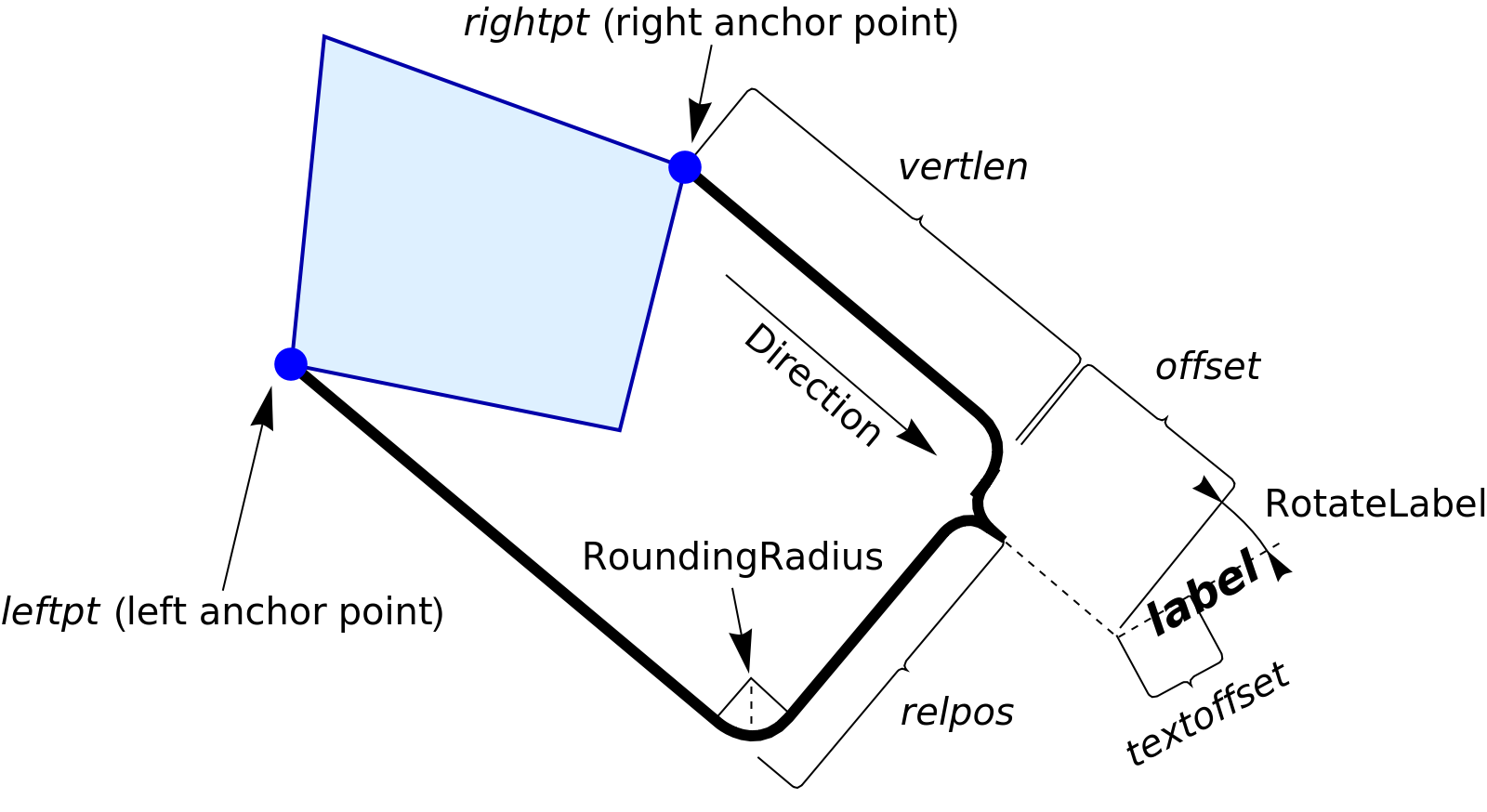The label offset scales with the size of Graphics to preserve the relative position of the label and the brace.
The anchor points can be specified either as Point objects or as {x,y} coordinate pairs.
When the first argument is set to Nothing, None or Missing[], no label is produced.
The following options can be given:
 RotateLabel True whether to rotate the label; if so, how much RoundingRadius 0.02 rounding radius of the joints of the brace curve Direction Automatic direction in which the edges extend from the anchor points
The option RotateLabel can be set to the following values:
 whether to rotate the label or not α rotate the label through a fixed angle {False,α} rotate the label through a fixed angle {True,α} add a fixed angle to the automatically calculated rotation
The option RoundingRadius can be set to the following values:
 r set the radius of the joints {rout,rin} set the radius of the outer joints and the inner joints, i.e. the cusp, independently
The option Direction can be set to the following values:
 Automatic adjust the direction according to the anchor points α direction given by an angle {x,y} direction given by a vector

## Examples

### Basic Examples (7)

Annotate a graphical object with an underbrace:

 In:=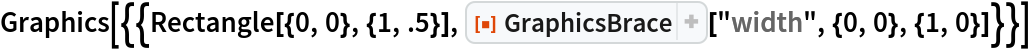Out=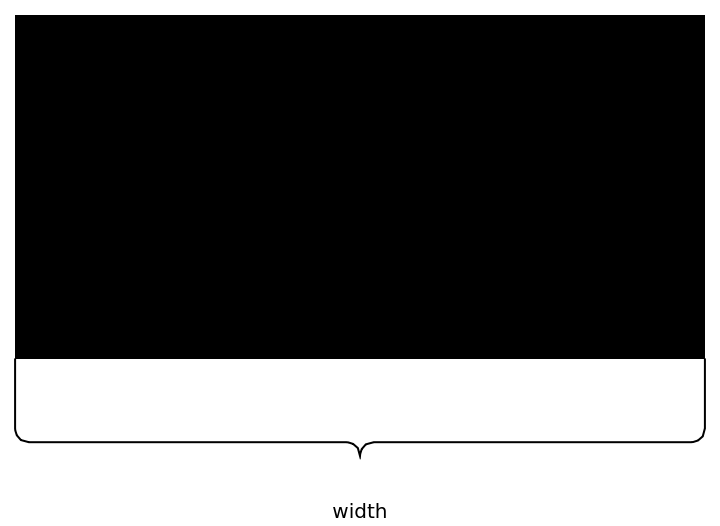Use multiple annotations:

 In:=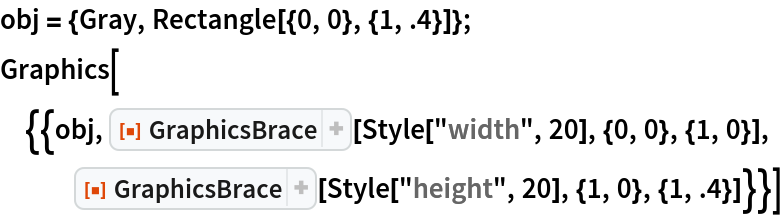Out=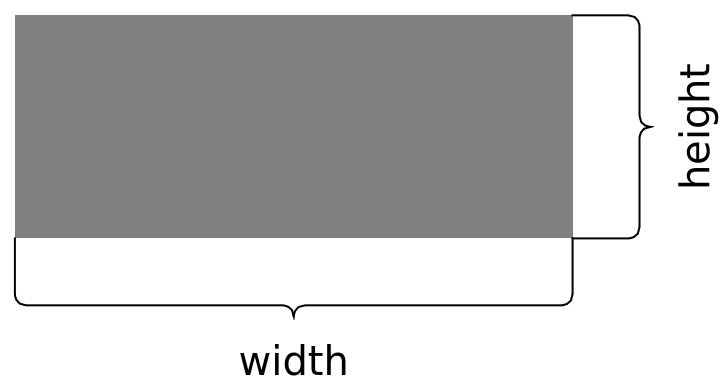By swapping the order of the two anchor points, create an overbrace:

 In:=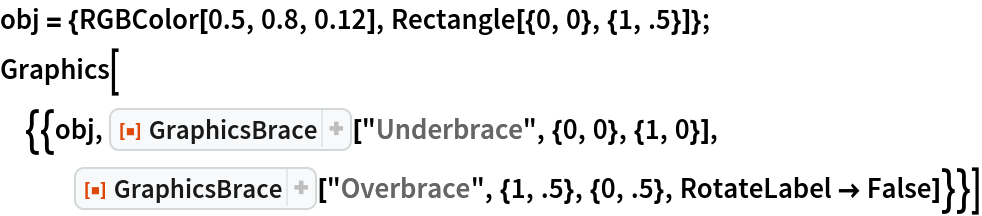Out=Adjust the position and rotation of the label:

 In:=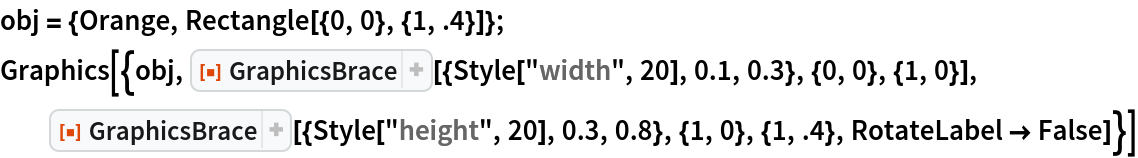Out=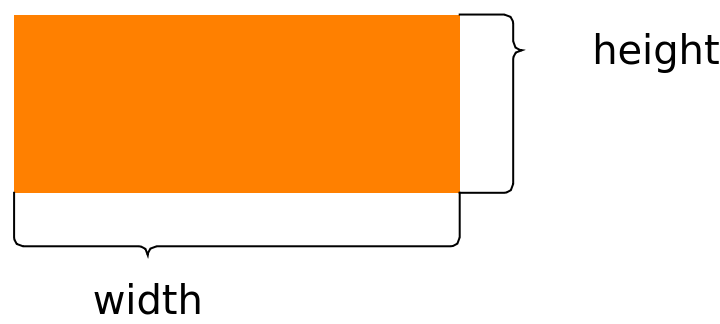By default, the brace automatically adjusts its rotation to the annotated object:

 In:=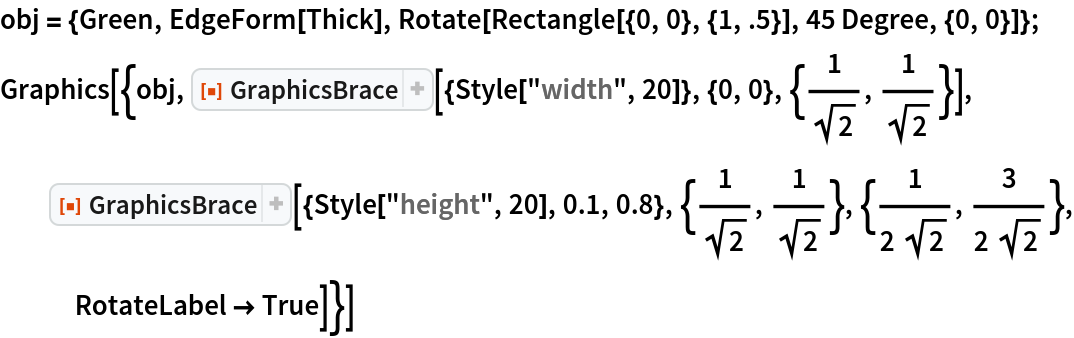Out=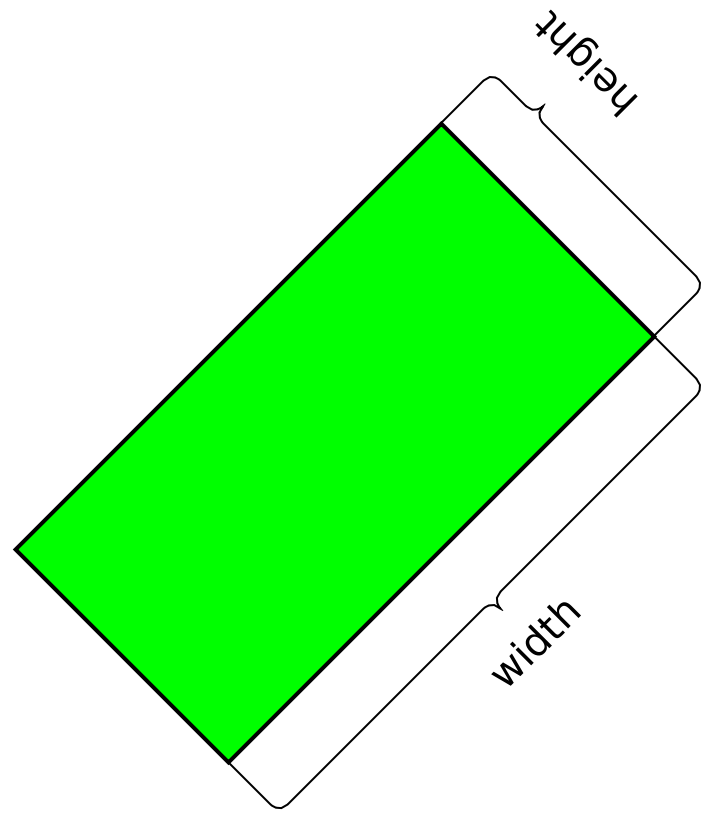Style the labels as well as the curves:

 In:=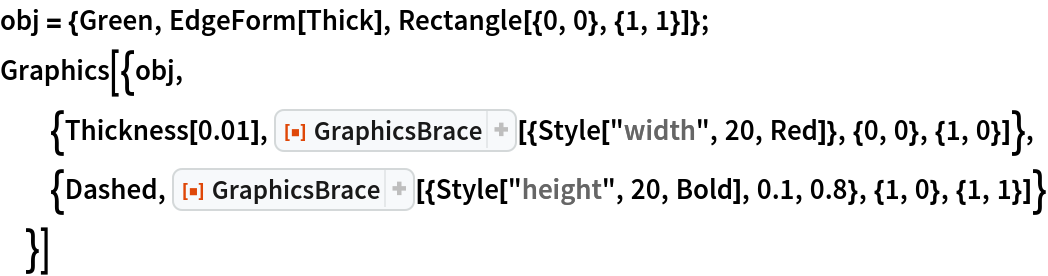Out=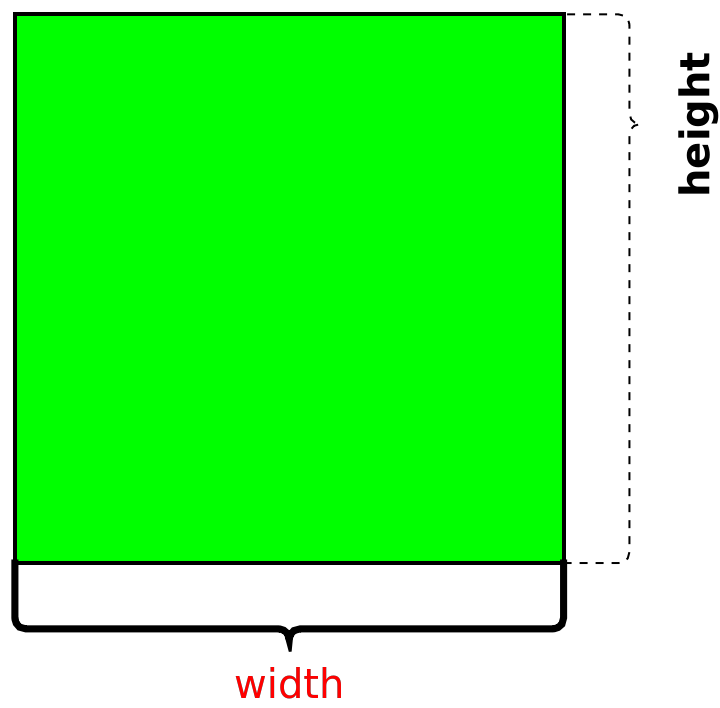Use Point objects directly as anchor points:

 In:=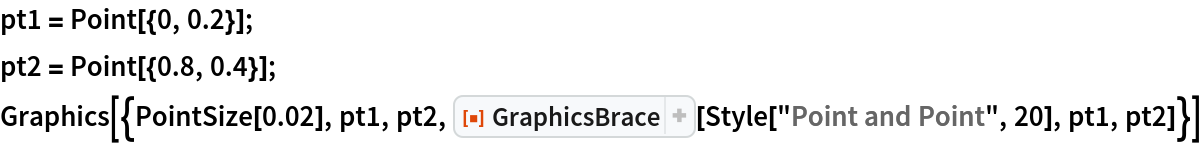Out=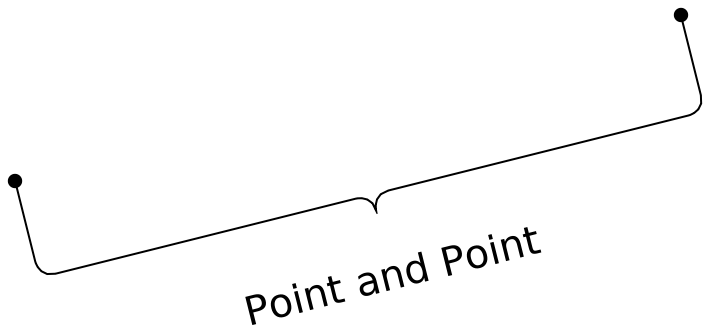### Scope (10)

#### Label content (2)

Use the underbrace with no label:

 In:=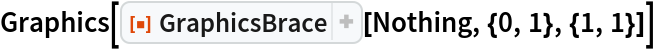Out=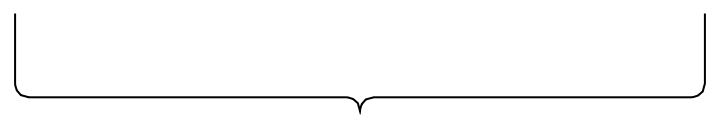The label does not have to be text:

 In:=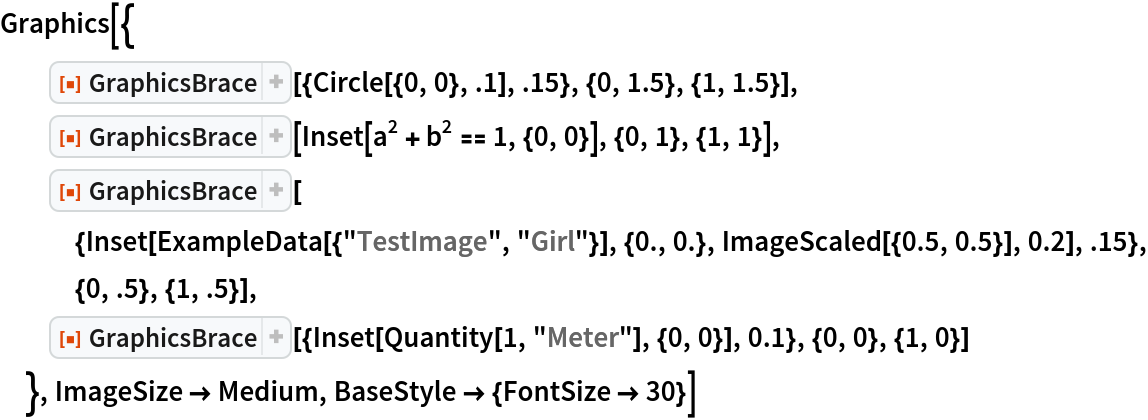Out=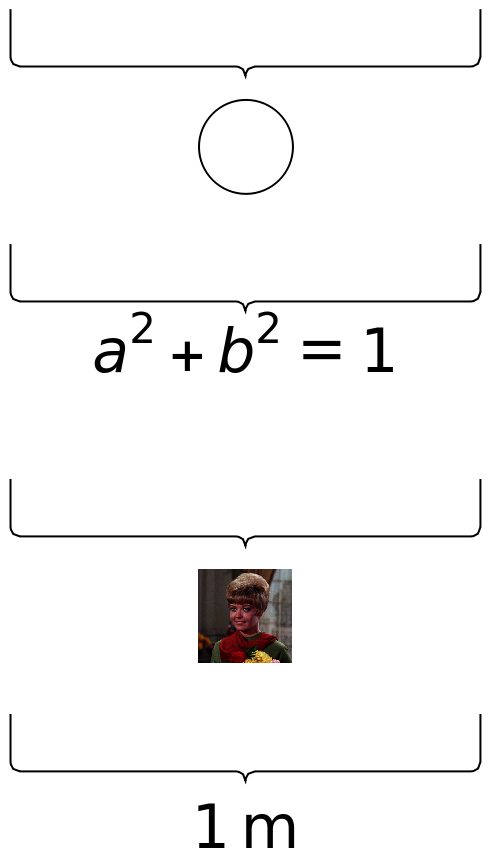#### Label position (3)

Position the label further from the cusp:

 In:=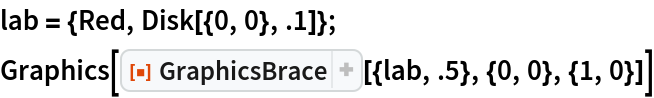Out=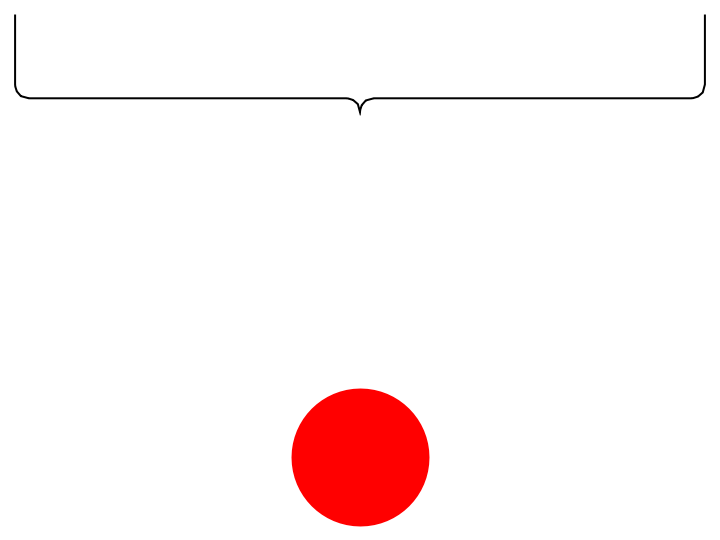The offset is taken in the direction of the cusp:

 In:=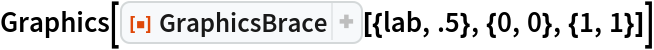Out=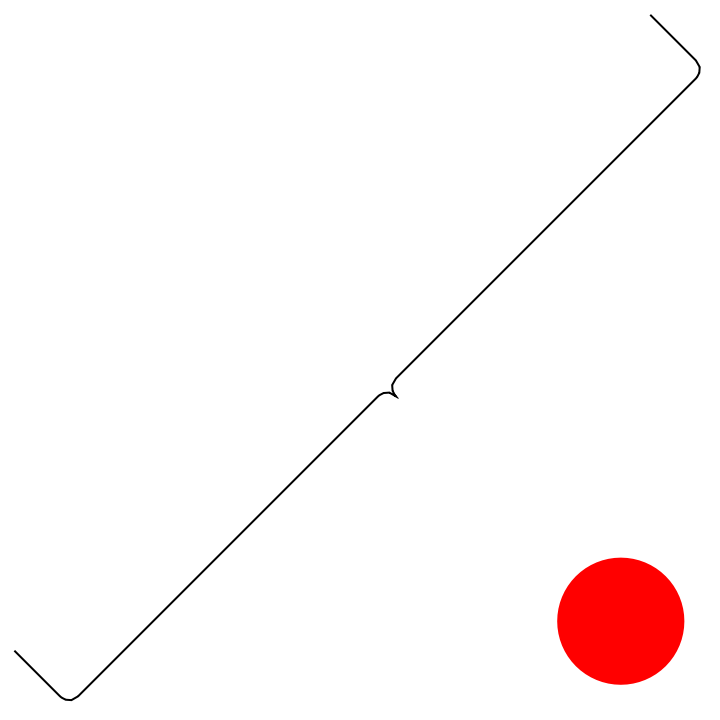The textual label can be offset in the horizontal and vertical directions using an additional argument:

 In:=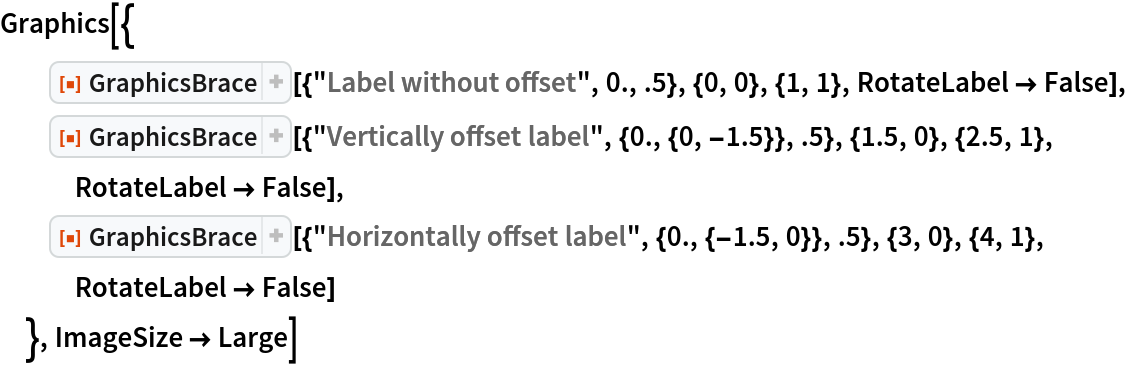Out=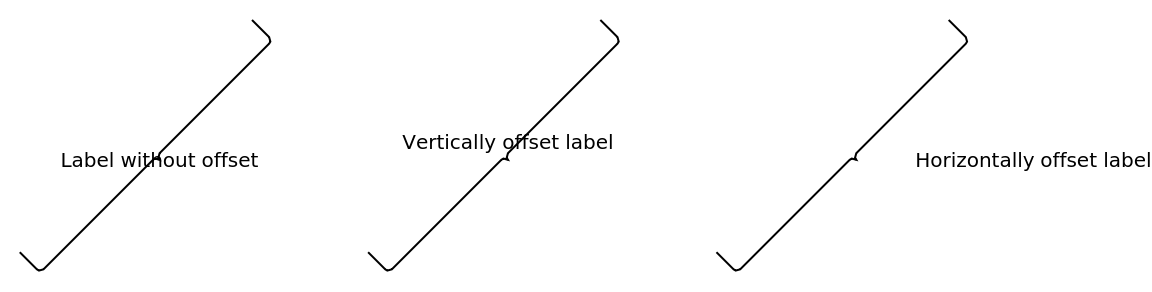Any specification that is valid for the third argument of Text is also allowed. Here, an Offset is used:

 In:=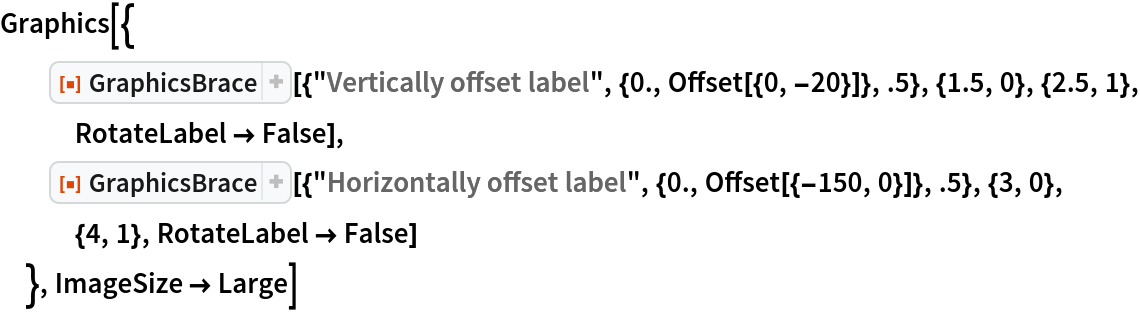Out=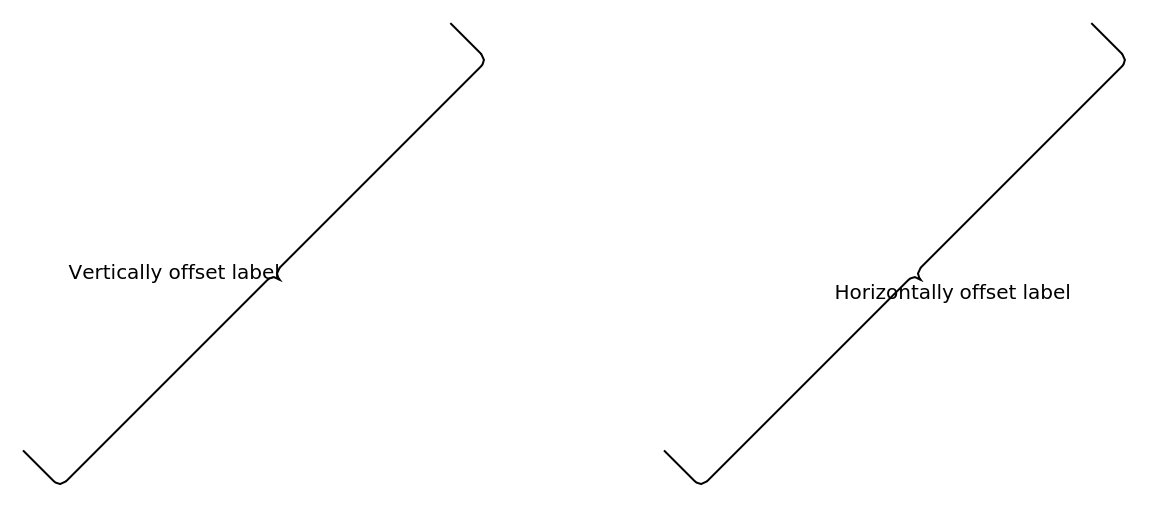Specify the relative position of the label with respect to the edges:

 In:=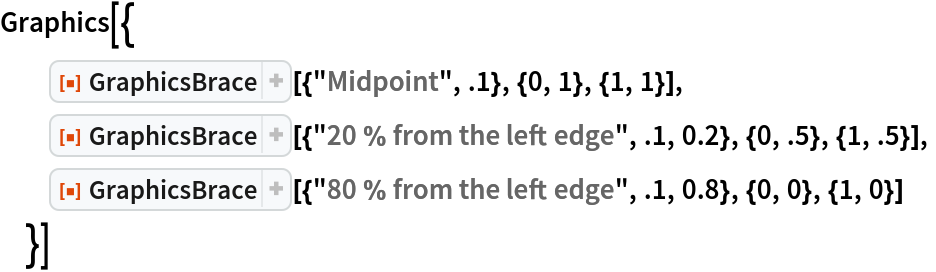Out=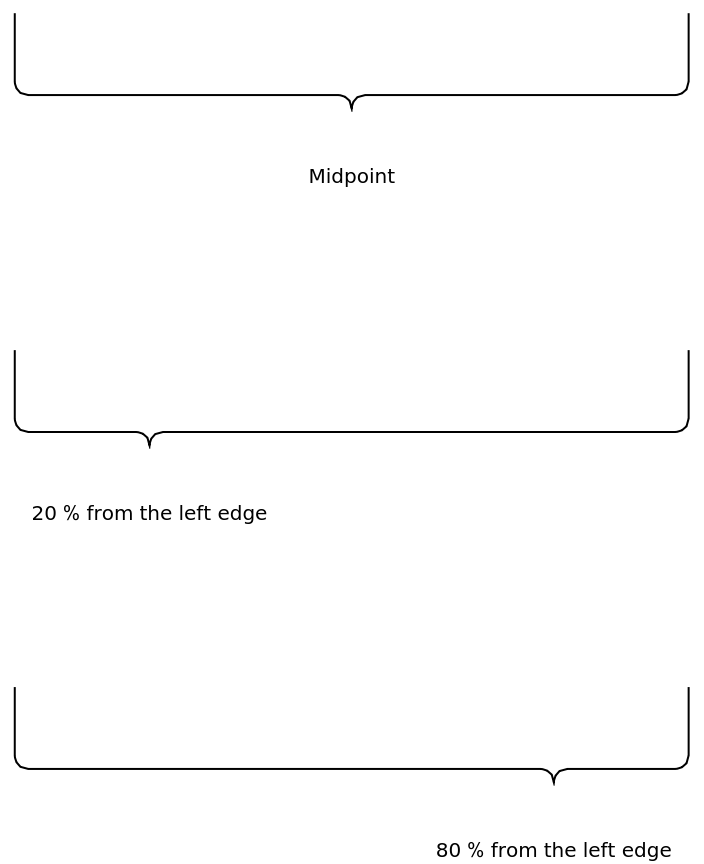#### Curve dimensions (2)

Make the edges longer:

 In:=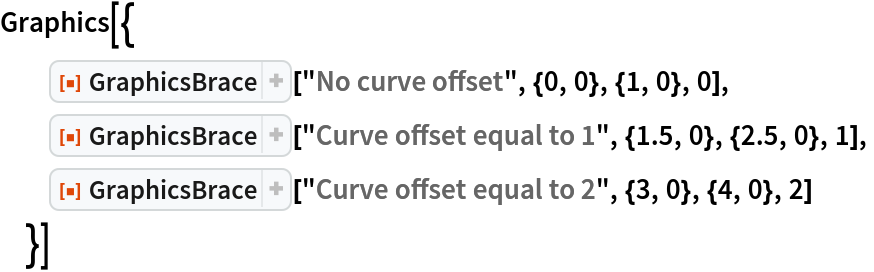Out=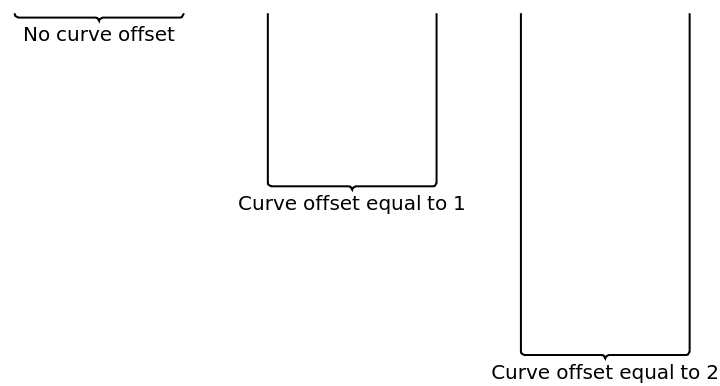Specify the relative position of the cusp with respect to the edges:

 In:=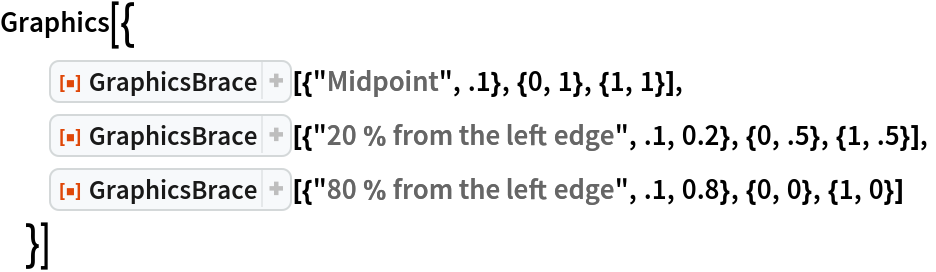Out=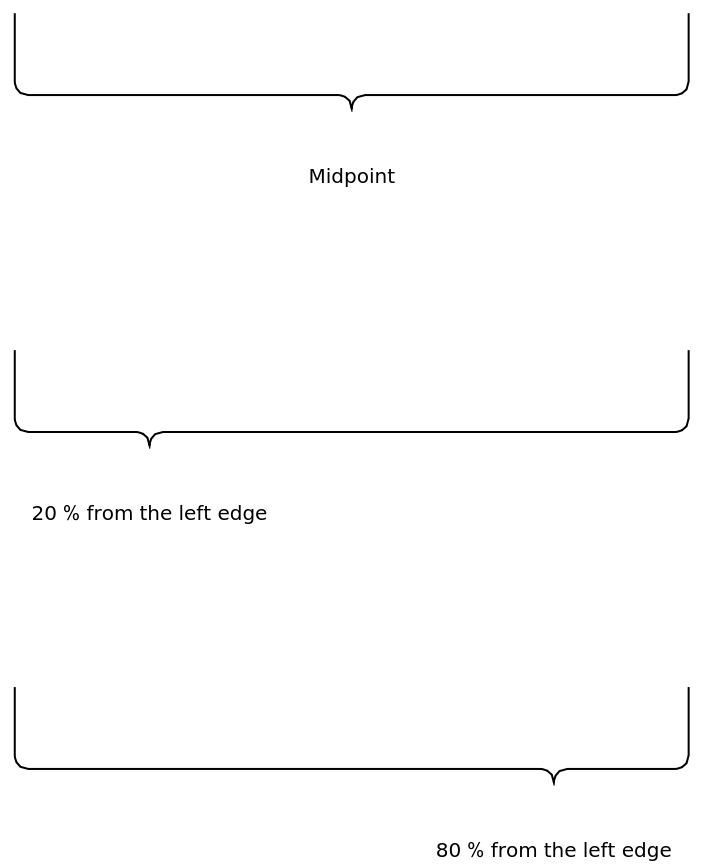#### Presentation (3)

Choose different colors, thicknesses, etc. for the curve:

 In:=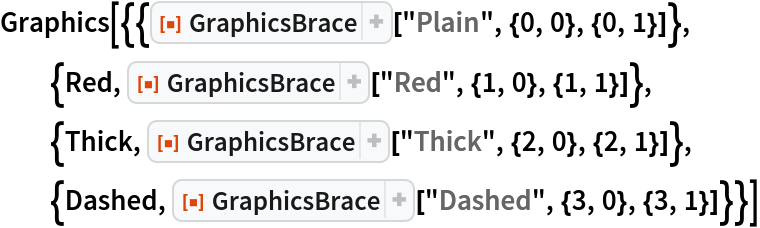Out=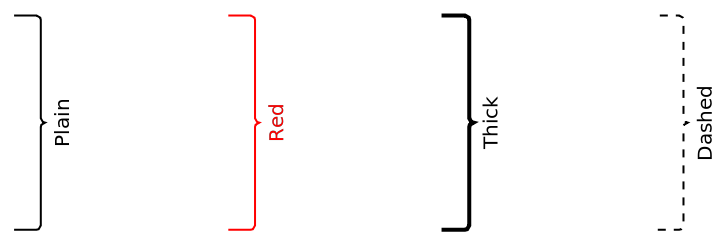Choose different styles for the label:

 In:=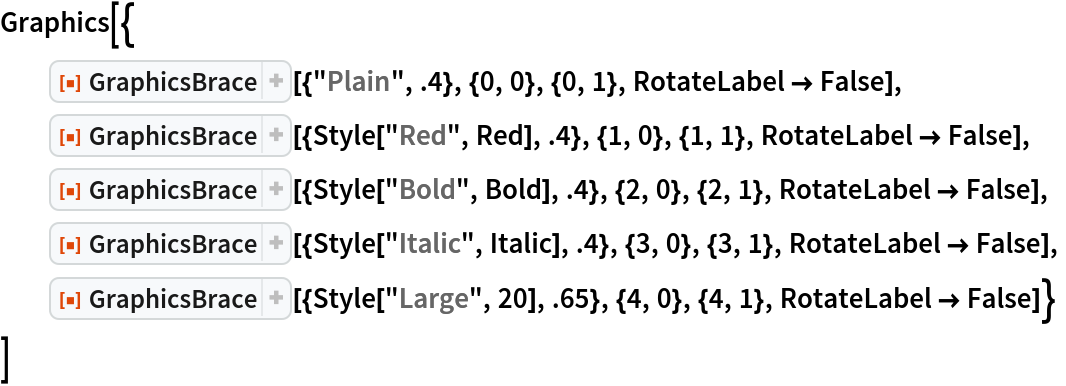Out=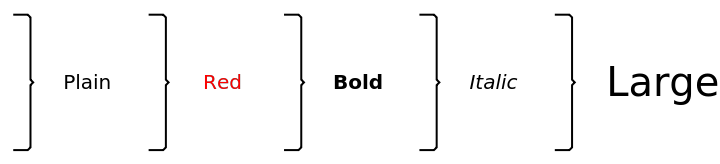Style both the curve and the label:

 In:=Out=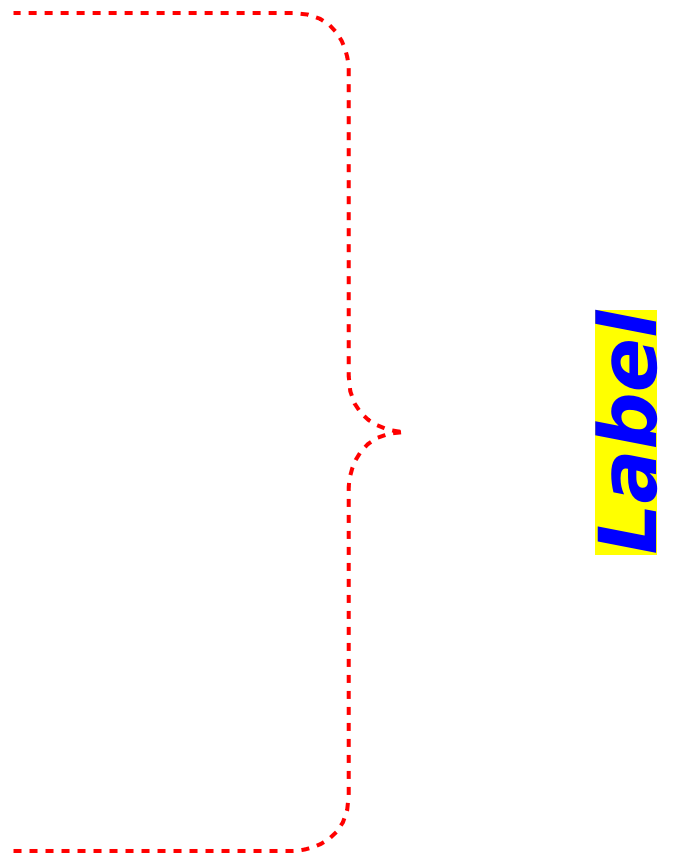### Options (8)

#### Direction (3)

By default, the curve stretches between the two anchor points:

 In:=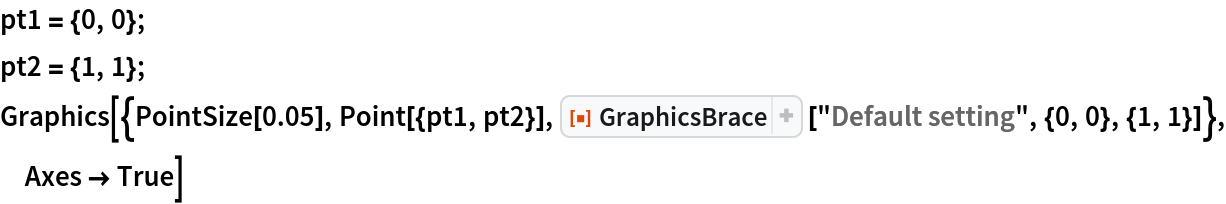Out=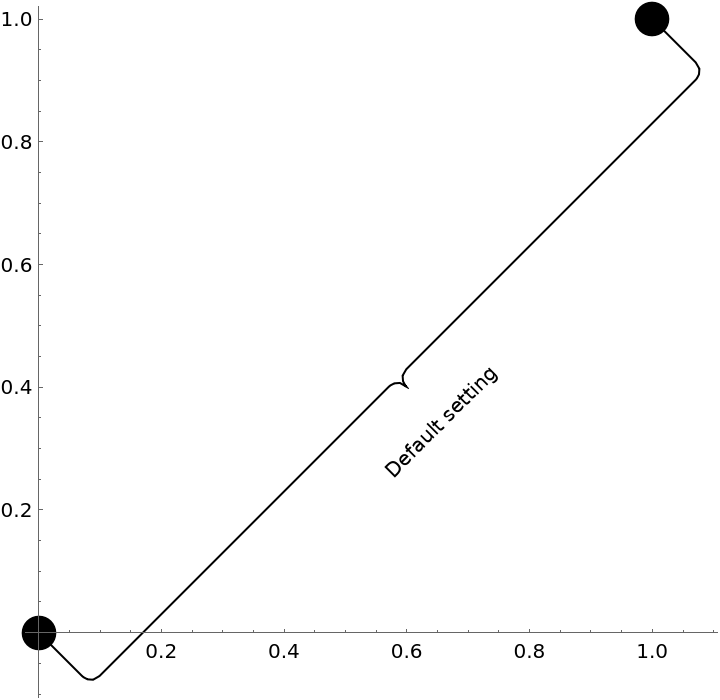Specify explicitly the direction of the edges:

 In:=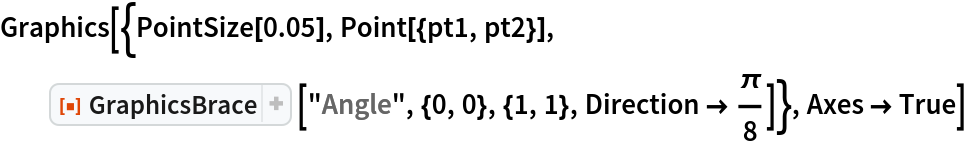Out=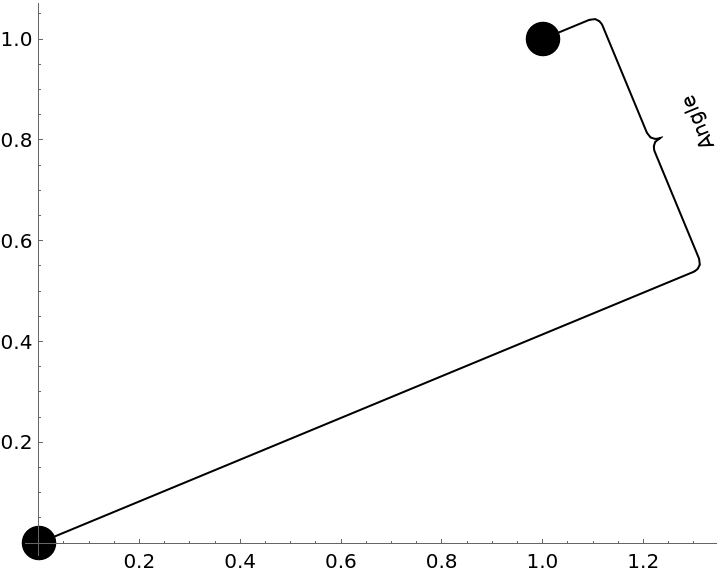The direction can also be specified as a vector:

 In:=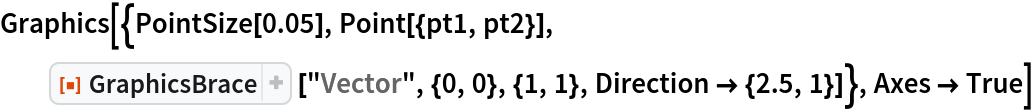Out=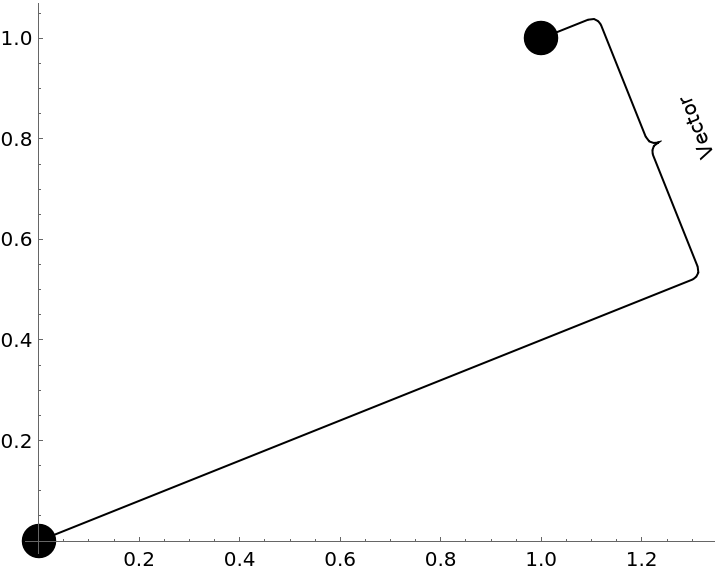#### RotateLabel (3)

The label is by default parallel to the brace:

 In:=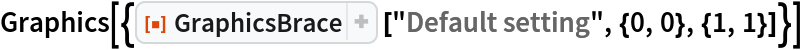Out=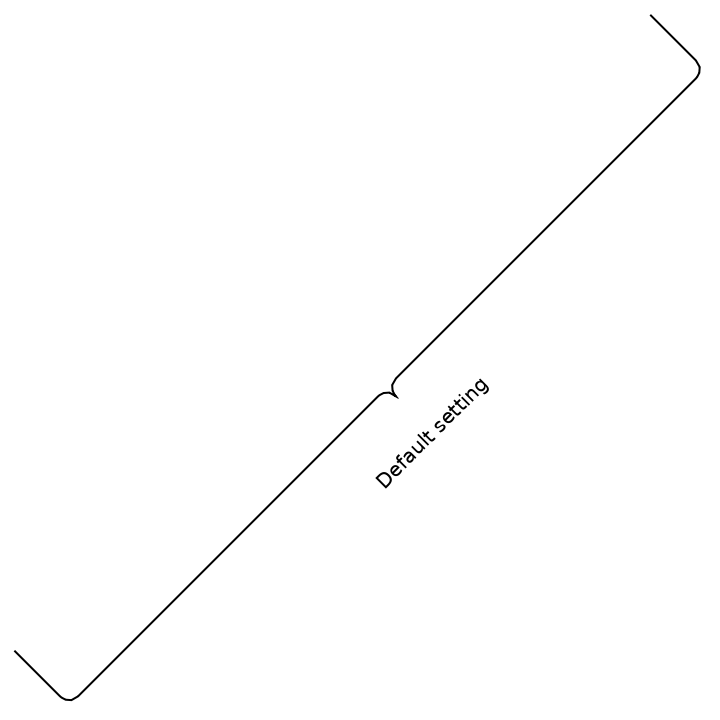Disable rotation:

 In:=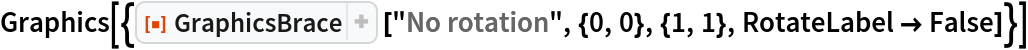Out=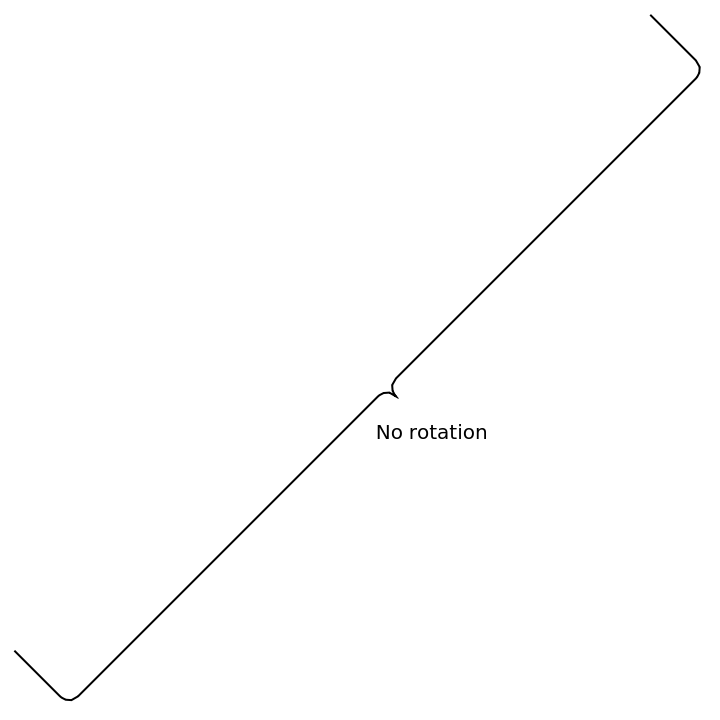The angle can also be given explicitly:

 In:=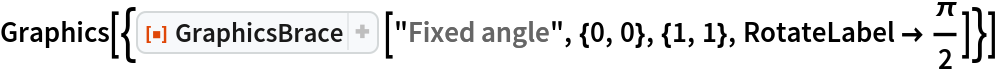Out=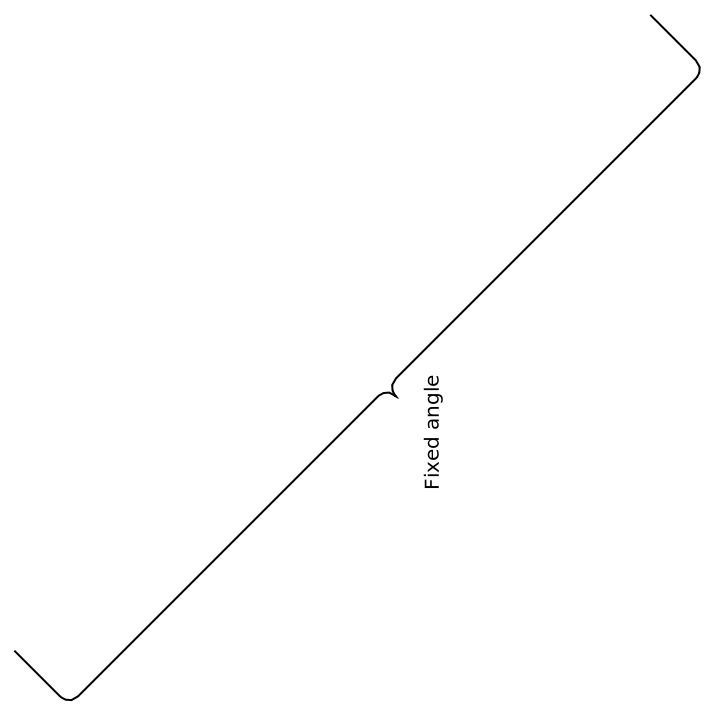The combination of both approaches yields an automatically rotated label with the explicitly given rotation offset:

 In:=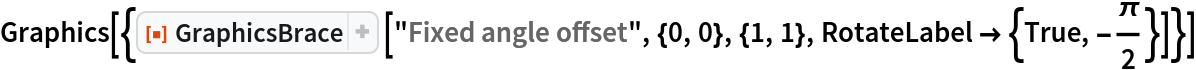Out=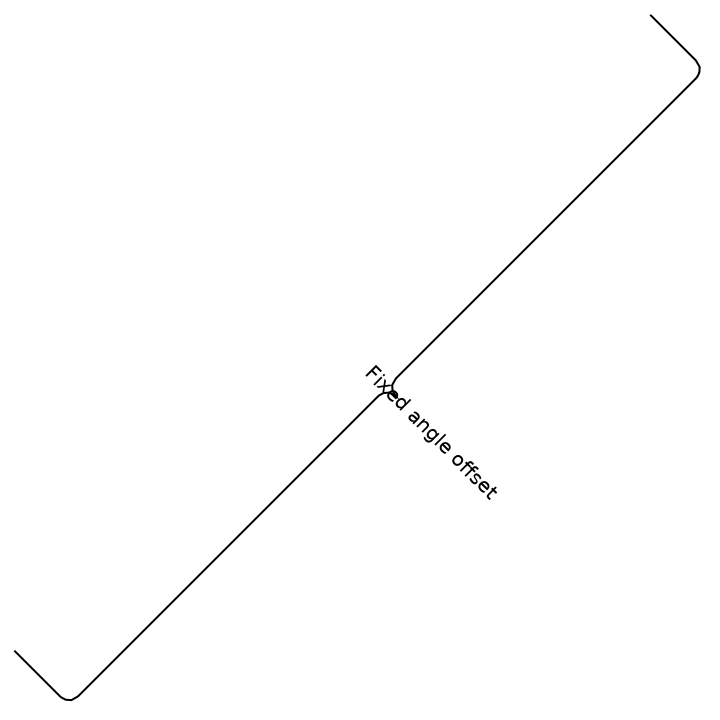In:=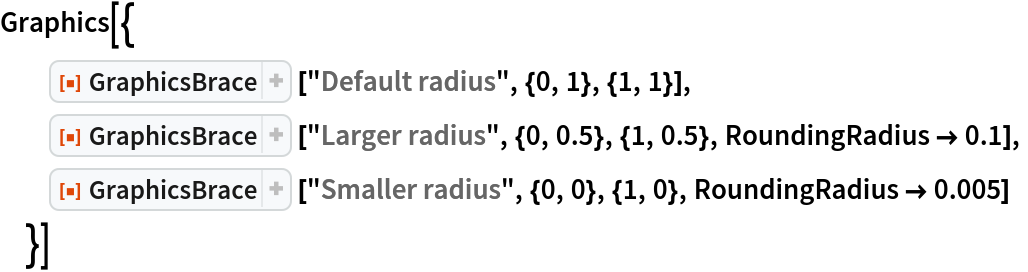Out=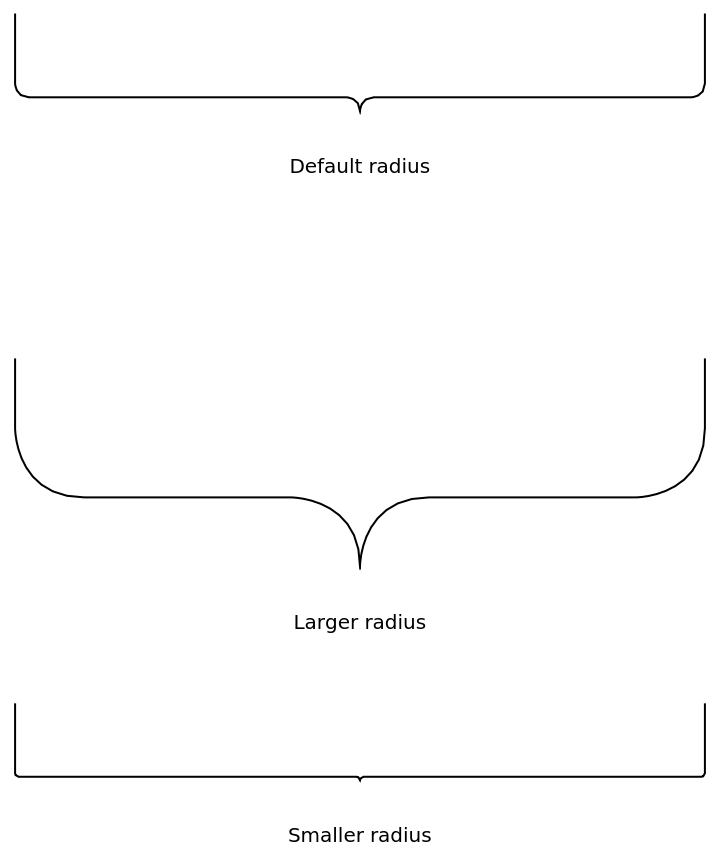The radius of the cusp and outer joints can be specified independently:

 In:=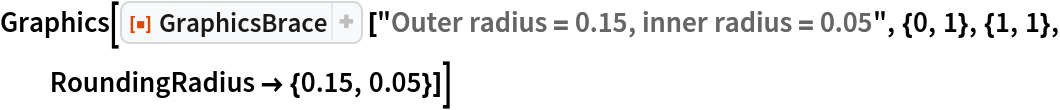Out=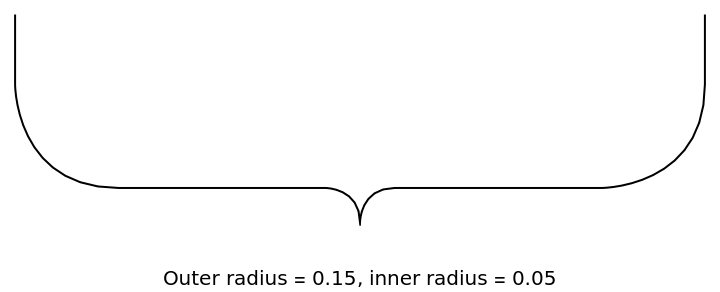### Applications (2)

Make a technical drawing with proper physical units:

 In:=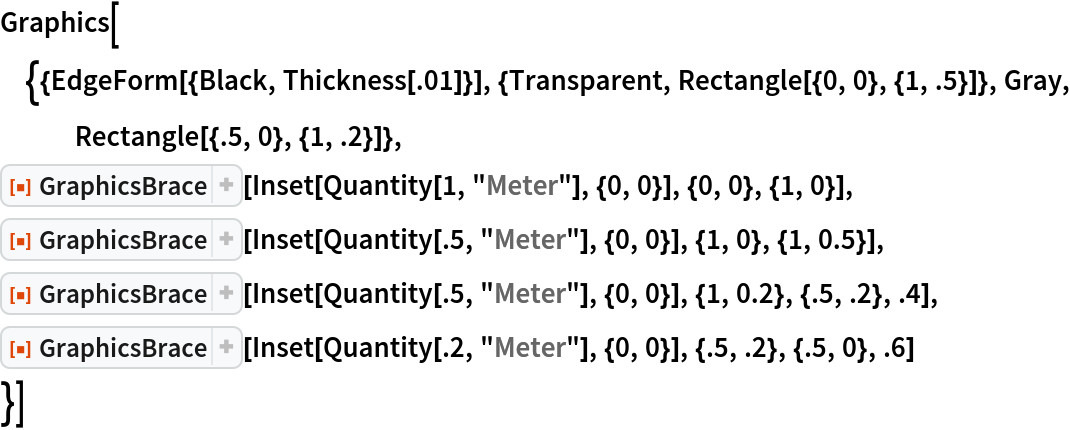Out=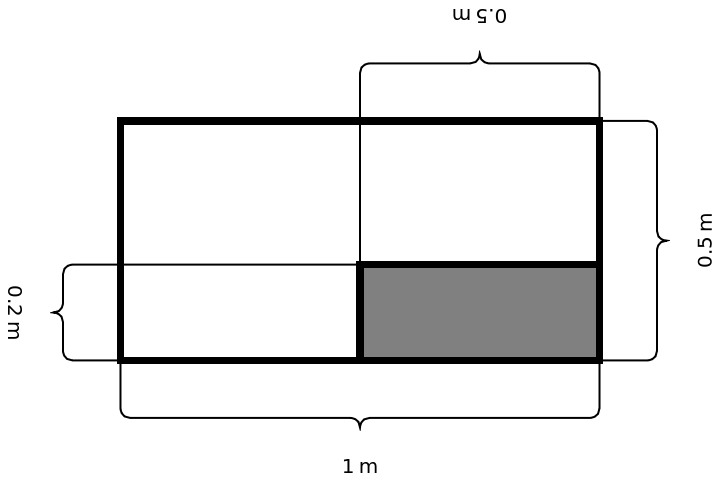Describe the regions where a given function is monotonically increasing and decreasing:

 In:=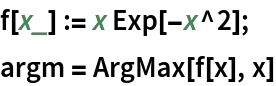Out=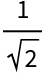In:=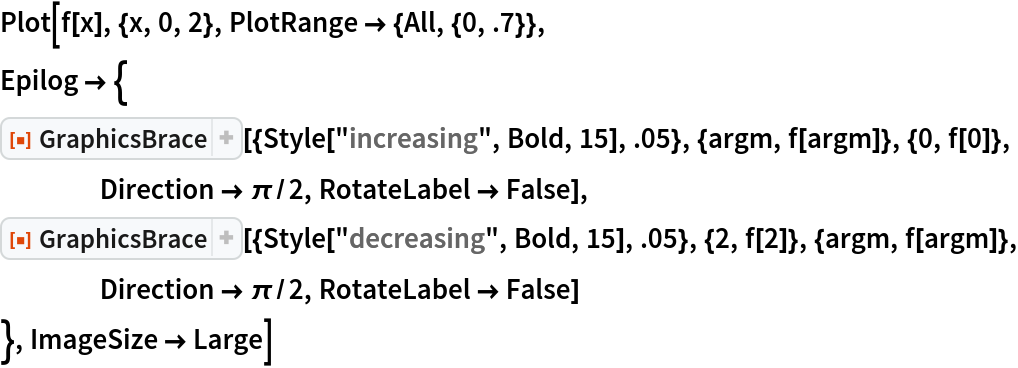Out=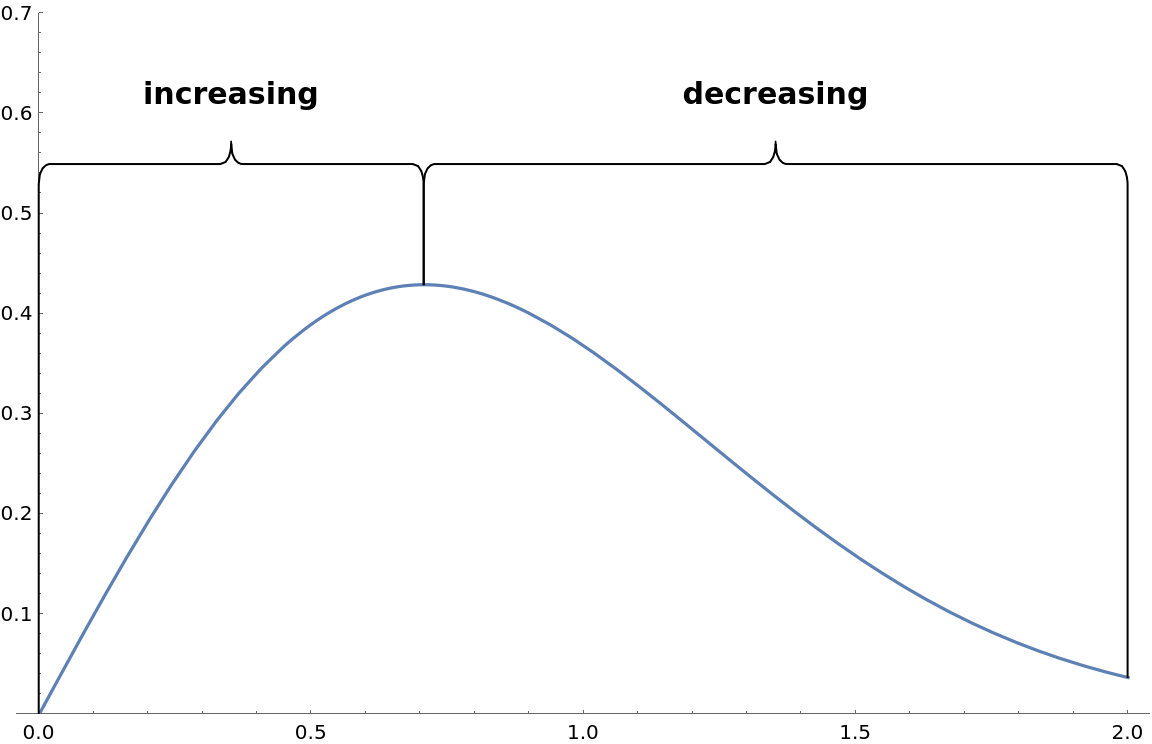### Properties and Relations (1)

GraphicsBrace can be used in Graphics the way that an underbrace is used in text:

 In:=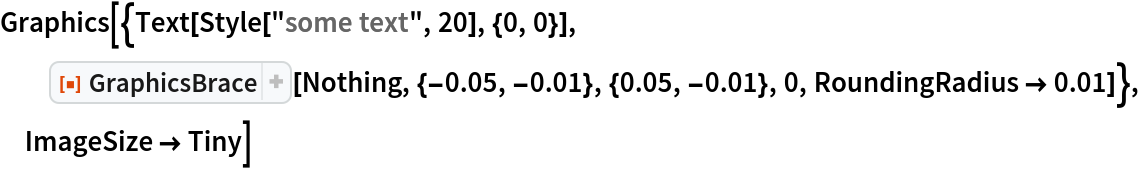Out=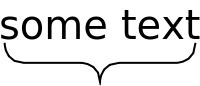### Possible Issues (5)

When the first argument is to be taken as a literal, wrap it in Inset:

 In:=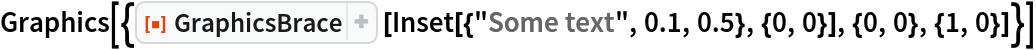Out=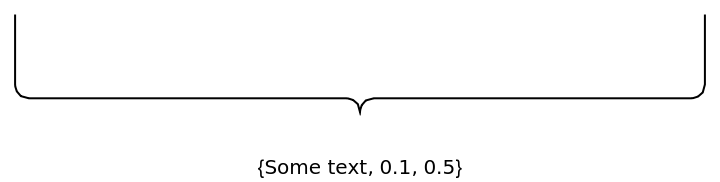When the other arguments are not numeric, no evaluation of GraphicsBrace takes place:

 In:=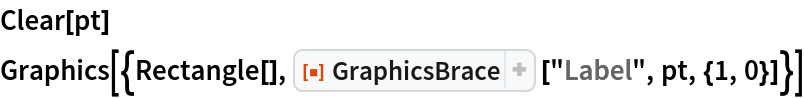Out=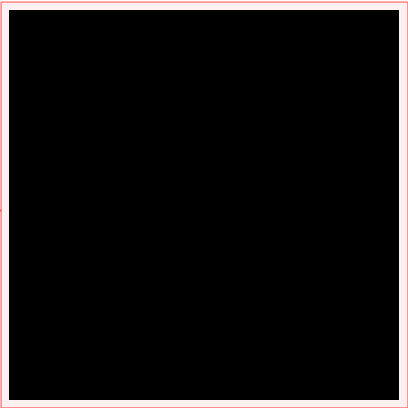In:=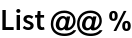Out=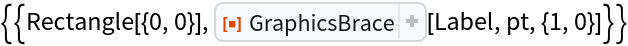The label may be set too close to the cusp:

 In:=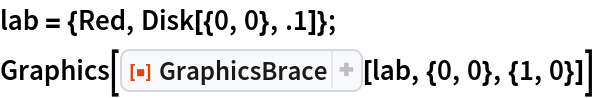Out=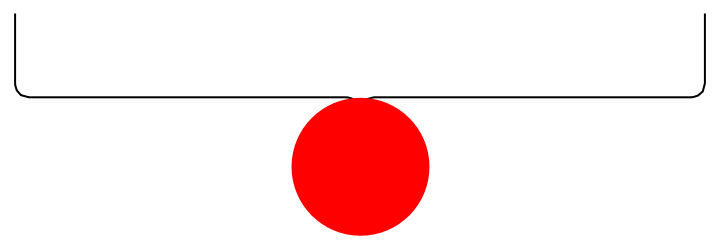Position the label further from the cusp:

 In:=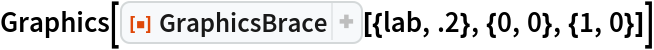Out=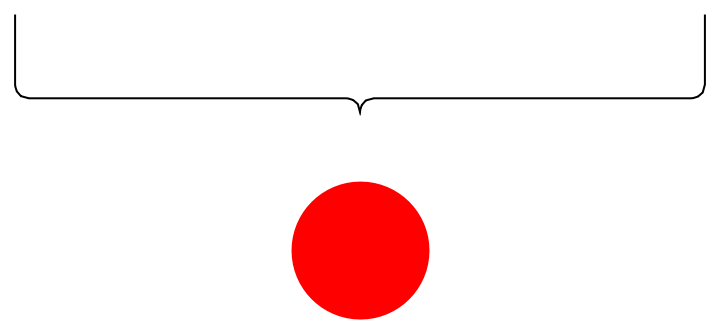When the line segments are too small with respect to the rounding radii, undesired behavior occurs:

 In:=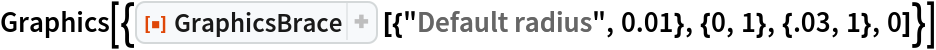Out=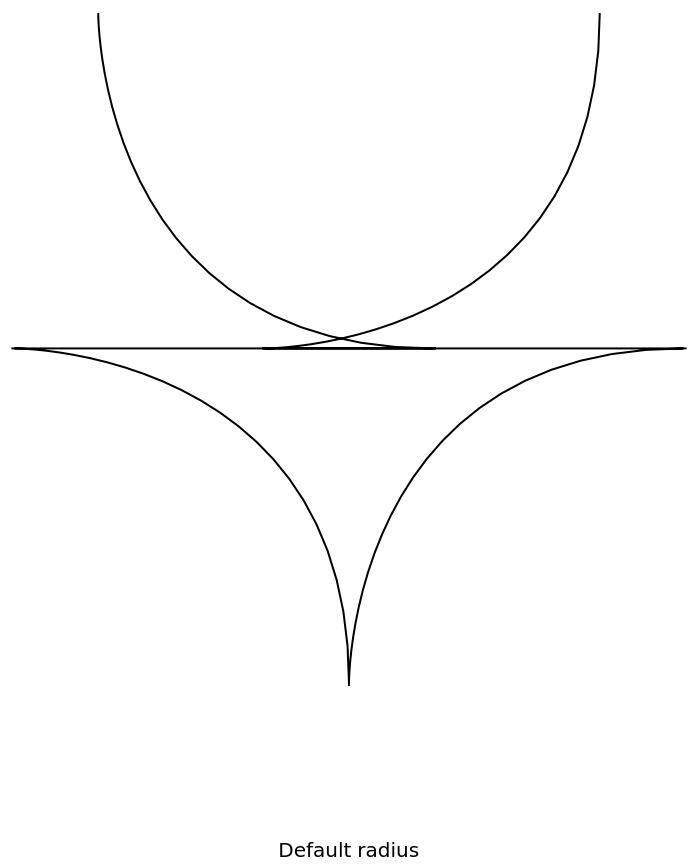In:=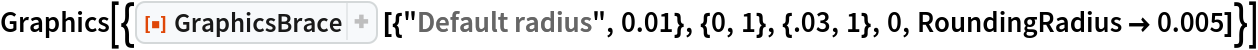Out=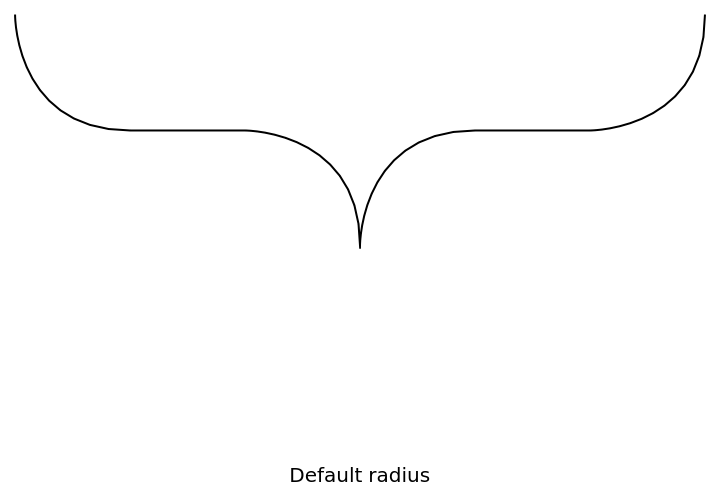For an overbrace, the label is upside down:

 In:=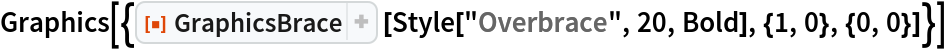Out=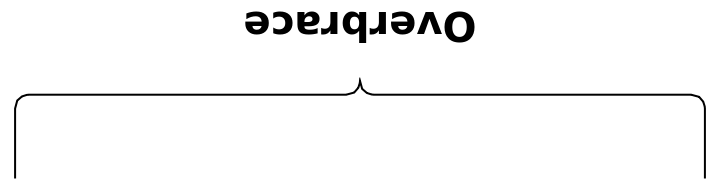Turn off the automatic rotation of the label:

 In:=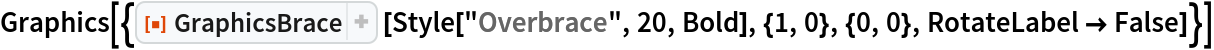Out=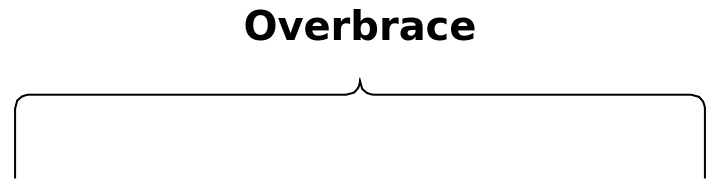### Neat Examples (2)

Use the brace as a graphics primitive for artistic drawing:

 In:=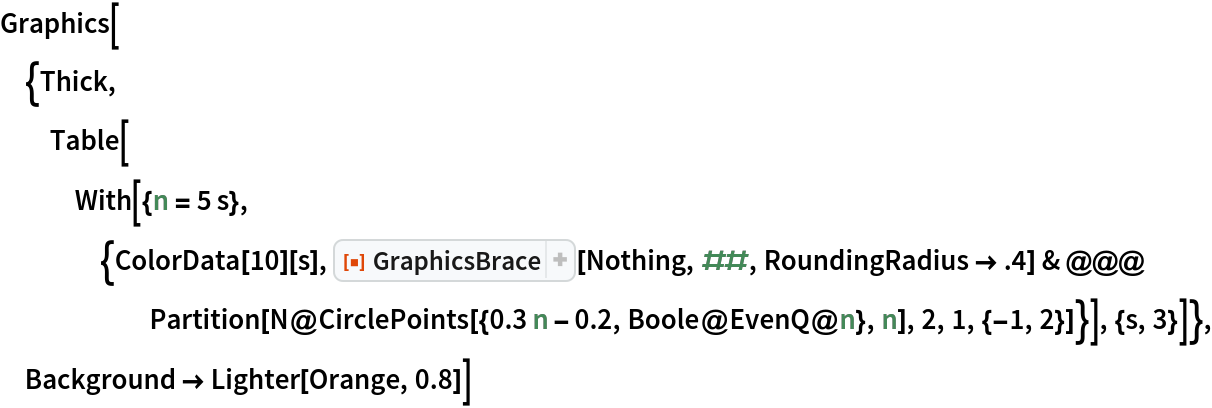Out=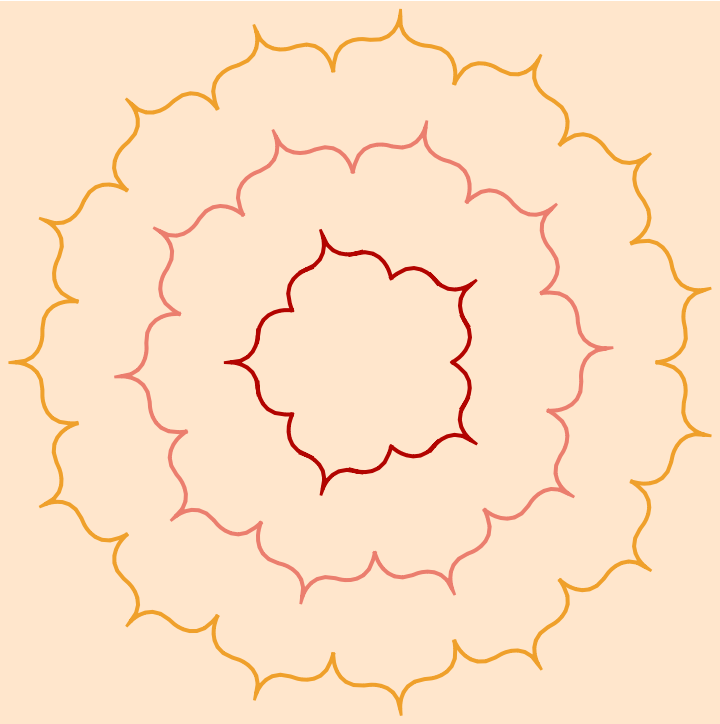Annotate the side lengths of a dynamically changing triangle:

 In:=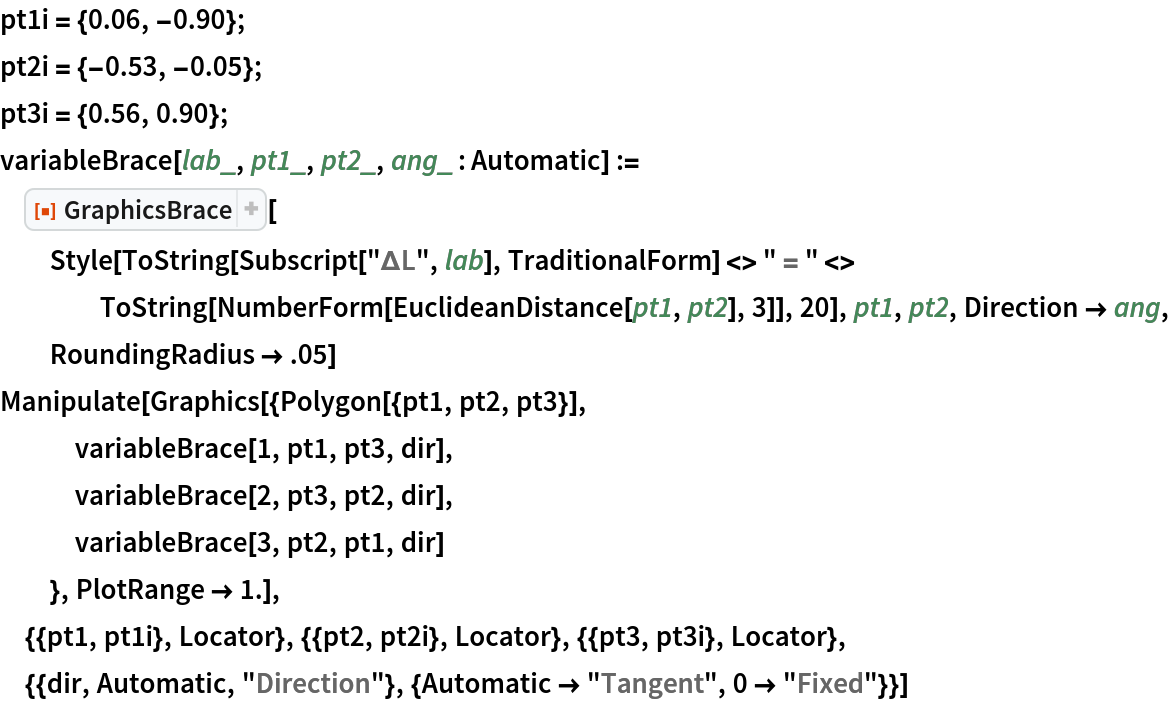Out=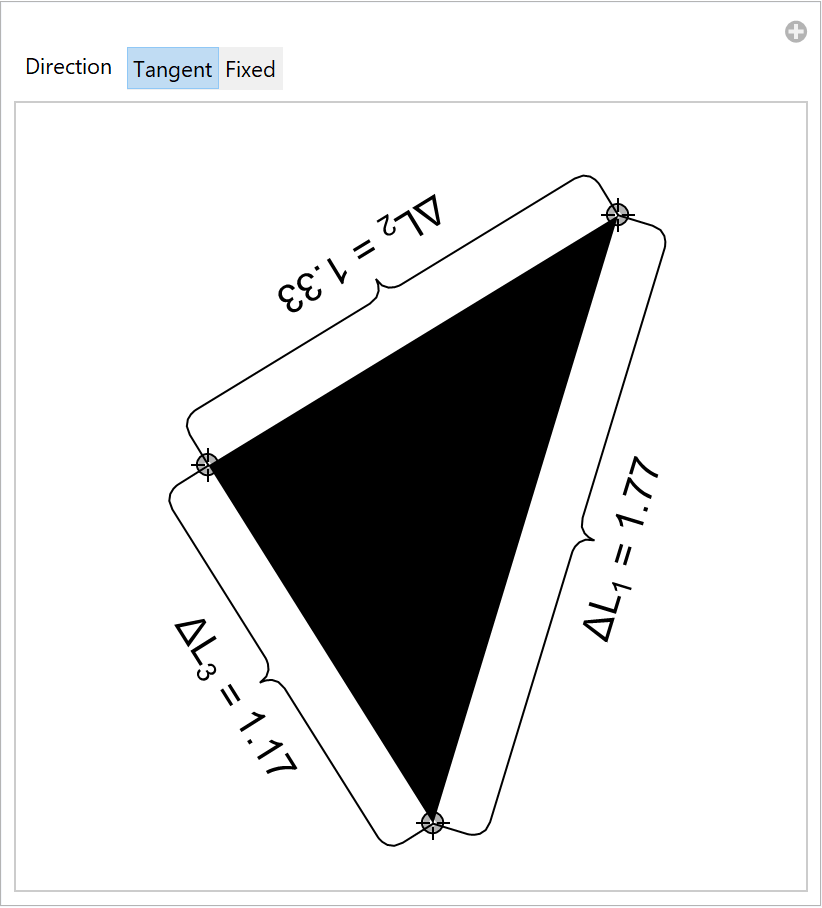Jaroslav Kysela

## Version History

• 1.0.0 – 26 May 2021